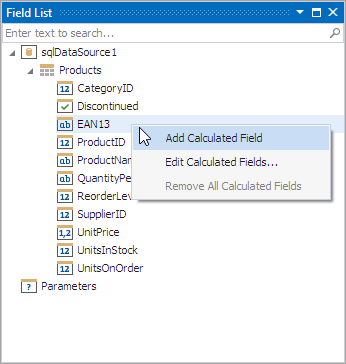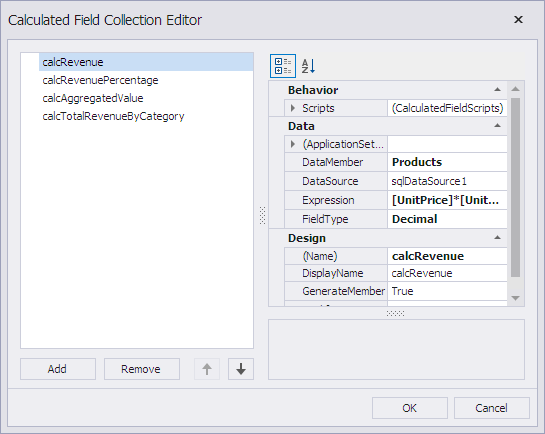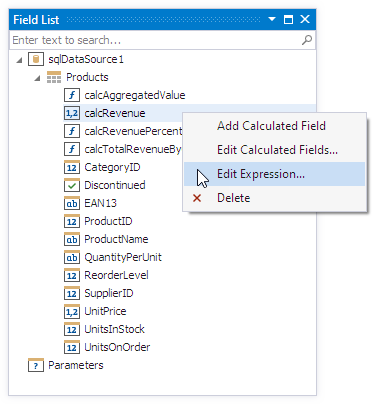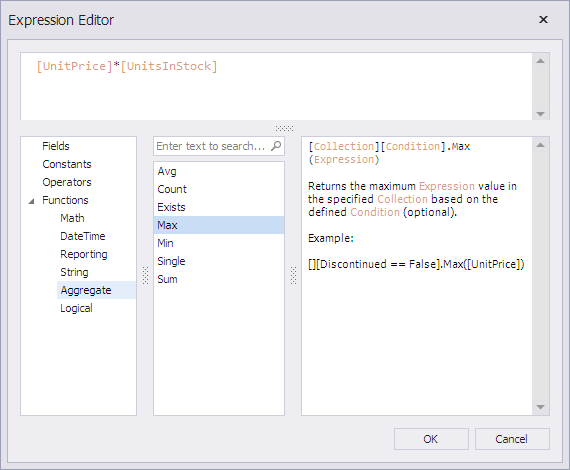# Calculated Fields

A calculated field is a field that calculates its value with an expression that may include existing database fields. A calculated field allows you to create new data that is not stored in the database, or select a value from the database fields based on certain criteria.

## Overview

Calculated fields are CalculatedField class instances. The XtraReport.CalculatedFields property returns the CalculatedFieldCollection that contains all calculated fields in the report.

To add a calculated field in the Report Designer, invoke the Field List window, right-click any field, and select Add Calculated Field:A new calculated field is created with the calculatedField1 default name.

You can select Edit Calculated Fields in the context menu to invoke the Calculated Field Collection Editor:The field type can be set explicitly. The specified field type determines how a calculated field is processed in expressions. The field type affects the field drag-and-drop behavior in the Field List dialog.

Note

The data source and data member settings are not automatically updated when you change the report data source. You should change them manually. For this, in the report’s Properties window, click the ellipsis next to the Calculated Fields property to invoke the Calculated Field Collection Editor and specify the data source and data member settings.

A calculated field evaluates its expression to calculate its value. Use the CalculatedField.Expression property to specify the field’s expression. To specify an expression in the Report Designer, invoke the Field List window, right-click the required field, and select Edit Expression to invoke the Expression Editor, which allows you to visually construct an expression:In an expression, you can use data fields, report parameters, predefined constants, and various date-time, logical, math, and string functions. The Expression Editor displays only data fields from a data source specified by the CalculatedField.DataSource and CalculatedField.DataMember property values.You can group and sort data in the report based on the calculated field values. Review the following topic, which contains an example of how to use a calculated field to group data: Group Data by Days of the Week.

Tip

If you cannot use calculated fields to accomplish your task, use the XRControl.EvaluateBinding event.

## Expression Syntax

A data field is defined by its name in square brackets:

``````[UnitPrice]
``````

A parameter has a question mark prefix (“?“) before its name:

``````?paramCompany
``````

A calculated field expression can include other calculated fields if you avoid cyclic references.

Date-time constants must be wrapped in hash symbols (#):

``````[OrderDate] >= #1/1/2020#
``````

Use a question mark to specify a null reference that does not refer to any object:

``````[Region] != ?
``````

Use apostrophes () to denote strings. To insert an apostrophe in the text of an expression, precede it with another apostrophe:

``````'It''s sample text'
``````

The type of a calculated field’s value is defined by the CalculatedField.FieldType property. If a calculated field expression involves different value types, use a function to convert them to the same type:

``````Max(ToDecimal([Quantity]),[UnitPrice])
``````

For more information on expression syntax, review the following help topic: Expression Language.

## Calculated Field Evaluation

For tasks that cannot be accomplished with the standard set of functions, you can evaluate your own function in the CalculatedField.GetValue event handler (or in its scripting counterpart).

Review the following help topic for the code sample: Sort Data by Custom Criteria.

## Create Calculated Field at Runtime

This example demonstrates how to create a calculated field at runtime, and bind the field to the report control’s Text property.

``````using DevExpress.XtraReports.UI;
using DevExpress.XtraReports.Configuration;
// ...
// Create a report.
XtraReport1 report = new XtraReport1();

// Create a calculated field
// and add it to the report's collection.
CalculatedField calcField = new CalculatedField();

// Specify the calculated field's properties.
calcField.DataSource = report.DataSource;
calcField.DataMember = report.DataMember;
calcField.FieldType = FieldType.Double;
calcField.DisplayName = "Calculated Field";
calcField.Name = "myField";
calcField.Expression = "[UnitPrice] * [UnitsInStock]";

// Bind the label's Text property to the calculated field.
report.FindControl("xrlabel3", true).ExpressionBindings